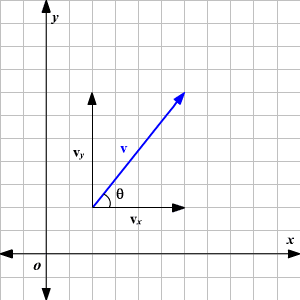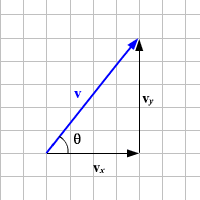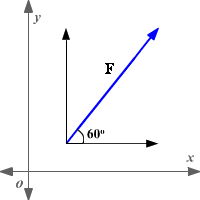# Components of a Vector

In a two-dimensional coordinate system, any vector can be broken into $x$ -component and $y$ -component.

$\stackrel{\to }{v}=⟨{v}_{x},{v}_{y}⟩$

For example, in the figure shown below, the vector $\stackrel{\to }{v}$ is broken into two components, ${v}_{x}$ and ${v}_{y}$ . Let the angle between the vector and its $x$ -component be $\theta$ .The vector and its components form a right angled triangle as shown below.In the above figure, the components can be quickly read. The vector in the component form is $\stackrel{\to }{v}=⟨4,5⟩$ .

The trigonometric ratios give the relation between magnitude of the vector and the components of the vector.

${v}_{x}=v\mathrm{cos}\theta$

${v}_{y}=v\mathrm{sin}\theta$

Using the Pythagorean Theorem in the right triangle with lengths ${v}_{x}$ and ${v}_{y}$ :

$|\text{\hspace{0.17em}}v\text{\hspace{0.17em}}|=\sqrt{{v}_{x}{}^{2}+{v}_{y}{}^{2}}$

Here, the numbers shown are the magnitudes of the vectors.

Case 1: Given components of a vector, find the magnitude and direction of the vector.

Use the following formulas in this case.

Magnitude of the vector is $|\text{\hspace{0.17em}}v\text{\hspace{0.17em}}|=\sqrt{{v}_{x}{}^{2}+{v}_{y}{}^{2}}$ .

To find direction of the vector, solve $\mathrm{tan}\theta =\frac{{v}_{y}}{{v}_{x}}$ for $\theta$ .

Case 2: Given the magnitude and direction of a vector, find the components of the vector.

Use the following formulas in this case.

${v}_{x}=v\mathrm{cos}\theta$

${v}_{y}=v\mathrm{sin}\theta$

Example:

The magnitude of a vector $\stackrel{\to }{F}$ is $10$ units and the direction of the vector is $60°$ with the horizontal. Find the components of the vector.$\begin{array}{l}{F}_{x}=F\mathrm{cos}60°\\ \text{\hspace{0.17em}}\text{\hspace{0.17em}}\text{\hspace{0.17em}}\text{\hspace{0.17em}}\text{\hspace{0.17em}}\text{\hspace{0.17em}}=10\cdot \frac{1}{2}\\ \text{\hspace{0.17em}}\text{\hspace{0.17em}}\text{\hspace{0.17em}}\text{\hspace{0.17em}}\text{\hspace{0.17em}}\text{\hspace{0.17em}}=5\end{array}$

$\begin{array}{l}{F}_{y}=F\mathrm{sin}60°\\ \text{\hspace{0.17em}}\text{\hspace{0.17em}}\text{\hspace{0.17em}}\text{\hspace{0.17em}}\text{\hspace{0.17em}}\text{\hspace{0.17em}}=10\cdot \frac{\sqrt{3}}{2}\\ \text{\hspace{0.17em}}\text{\hspace{0.17em}}\text{\hspace{0.17em}}\text{\hspace{0.17em}}\text{\hspace{0.17em}}\text{\hspace{0.17em}}=5\sqrt{3}\end{array}$

So, the vector $\stackrel{\to }{F}$ is $⟨5,5\sqrt{3}⟩$ .